Question

A diffraction grating has 3 x 106 lines per meter. The grating is illuminated by monochromatic plane waves of wavelength 600 nm at normal incidence that forms an interference pattern on a screen located 0.50 m away

a) Find the angle the first bright fringe.

b) Find the angle corresponding to the second bright fringe.

c) Find the angle of the third bright fringe.

slit width on grating (d) = 1/(3*!0^6) m

The equation for interfrence pattern dsin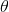= m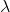a) For first bright fringe

dsin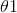= 1sin= 600*10^-9 /{1/3*10^6} = 1.8

Not a possible solution

b) for Second bright fringe

d sin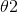= 2sin= 2* 600*10^-9 /{1/3*10^6} = 3.6

also not a possible solution

c)

for Second bright fringe

d sin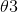= 2sin= 2* 600*10^-9 /{1/3*10^6} = 5.4

also not a possible solution.

For this grating interefence pattern does not form with this grating.

#### Earn Coins

Coins can be redeemed for fabulous gifts.

Similar Homework Help Questions
• ### A diffraction grating has 3 x 106 lines per meter. The grating is illuminated by monochromatic...

A diffraction grating has 3 x 106 lines per meter. The grating is illuminated by monochromatic plane waves of wavelength 600 nm at normal incidence that forms an interference pattern on a screen located 0.50 m away. a) Find the angle the first bright fringe. b) Find the angle corresponding to the second bright fringe. c) Find the angle of the third bright fringe.

• ### A diffraction grating has 3 x 106 lines per meter. The grating is illuminated by monochromatic...

A diffraction grating has 3 x 106 lines per meter. The grating is illuminated by monochromatic plane waves of wavelength 600 nm at normal incidence that forms an interference pattern on a screen located 0.50 m away. a) Find the angle the first bright fringe. b) Find the angle corresponding to the second bright fringe. c) Find the angle of the third bright fringe.

• ### Q. A diffraction grating has 3 x 106 lines per meter. The grating is illuminated by...

Q. A diffraction grating has 3 x 106 lines per meter. The grating is illuminated by monochromatic plane waves of wavelength 600 nm at normal incidence that forms an interference pattern on a screen located 0.50 m away a) Find the angle the first bright fringe. b) Find the angle corresponding to the second bright fringe. c) Find the angle of the third bright fringe.

• ### Q. A diffraction grating has 3 x 106 lines per meter. The grating is illuminated by...

Q. A diffraction grating has 3 x 106 lines per meter. The grating is illuminated by monochromatic plane waves of wavelength 600 nm at normal incidence that forms an interference pattern on a screen located 0.50 m away a) Find the angle the first bright fringe. b) Find the angle corresponding to the second bright fringe. c) Find the angle of the third bright fringe.

• ### 4. Light, with 550 nm wavelength, is used to illuminate double slits which are separated of...

4. Light, with 550 nm wavelength, is used to illuminate double slits which are separated of 3.5 x 10-5 m. A screen is placed 1.25 m from the slits.    a. Find the angle of the first bright fringe.    b. Find the angle of the third bright fringe.           A diffraction grating has 3 x 106 lines per meter. The grating is illuminated by monochromatic plane waves of wavelength 600 nm at normal incidence that forms an interference...

• ### 4. Light, with 550 nm wavelength, is used to illuminate double slits which are separated of...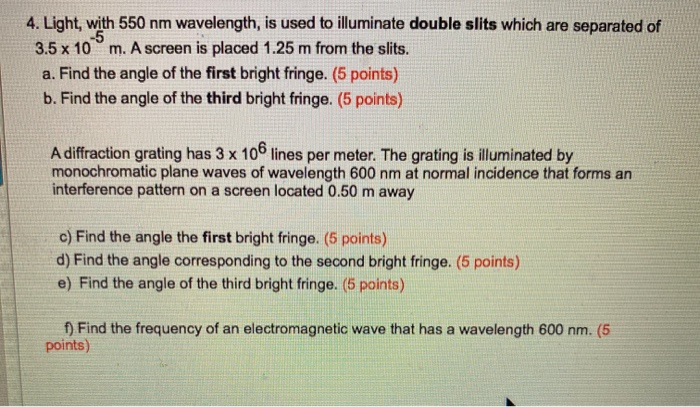4. Light, with 550 nm wavelength, is used to illuminate double slits which are separated of -5 3.5 x 10 m. A screen is placed 1.25 m from the slits. a. Find the angle of the first bright fringe. (5 points) b. Find the angle of the third bright fringe. (5 points) A diffraction grating has 3 x 106 lines per meter. The grating is illuminated by monochromatic plane waves of wavelength 600 nm at normal incidence that forms an...

• ### Monochromatic light shines on a diffraction grating with 8,600 lines uniformly distributed over 1.8 cm. The...

Monochromatic light shines on a diffraction grating with 8,600 lines uniformly distributed over 1.8 cm. The grating is illuminated using a laser of 630 nm wavelength. A diffraction pattern is formed on a screen located at 1.7 m away from the grating. (a) What is the angle of the first-order maximum of the 630-nm light incident upon the grating? (b) What is the separation on the screen between the first and the second order maxima? (c) What is the highest...

• ### 1. Monochromatic blue light of wavelength 440-nm passes through a 3300 lines/cm diffraction grating and the...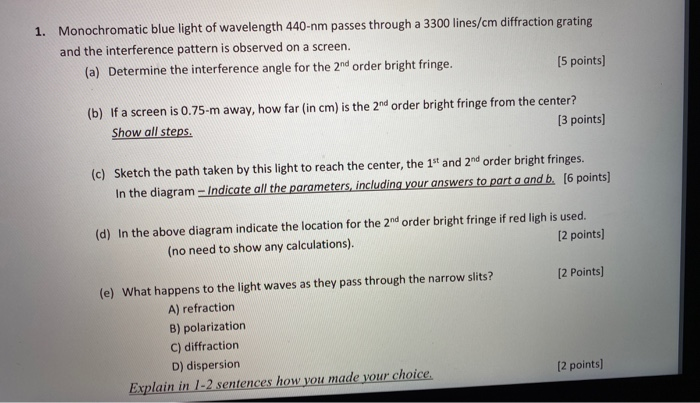1. Monochromatic blue light of wavelength 440-nm passes through a 3300 lines/cm diffraction grating and the interference pattern is observed on a screen. (a) Determine the interference angle for the 2nd order bright fringe. (5 points) (b) If a screen is 0.75-m away, how far (in cm) is the 2nd order bright fringe from the center? Show all steps. [3 points) (c) Sketch the path taken by this light to reach the center, the 1st and 2nd order bright fringes....

• ### 1. Monochromatic blue light of wavelength 440-nm passes through a 3300 lines/cm diffraction grating and the...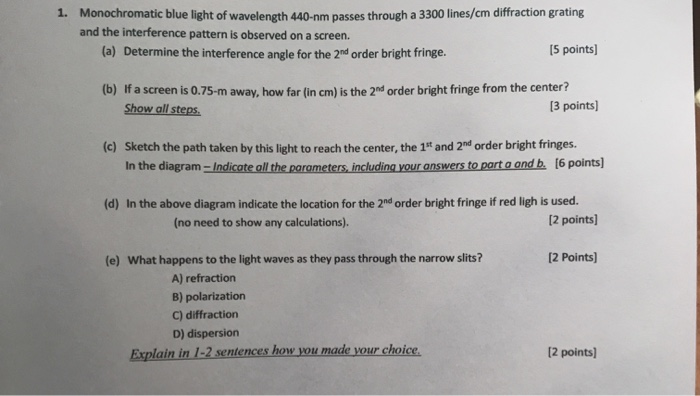1. Monochromatic blue light of wavelength 440-nm passes through a 3300 lines/cm diffraction grating and the interference pattern is observed on a screen. (a) Determine the interference angle for the 2nd order bright fringe. 15 points) (b) If a screen is 0.75-m away, how far (in cm) is the 2nd order bright fringe from the center? Show all steps. [3 points) (c) Sketch the path taken by this light to reach the center, the 1st and 2nd order bright fringes....

• ### Short Answer (6 points diffraction grating has 5150 lines diffraction per centimeter ruled on it. It...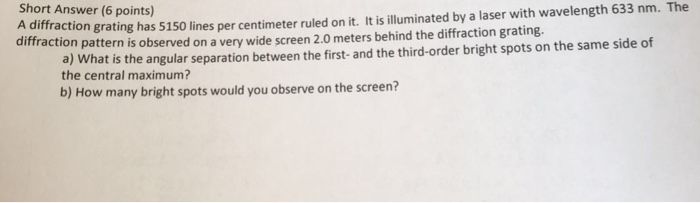Short Answer (6 points diffraction grating has 5150 lines diffraction per centimeter ruled on it. It is illuminated by a laser with wavelength 633 nm. The a) What is the angular separation between the first- and the third-order bright spots on the same side of the central maximum? pattern is observed on a very wide screen 2.0 meters behind the diffraction grating. b) How many bright spots would you observe on the screen?